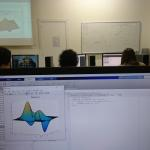# Modelling and management of wild populations

University
Teacher
Semester
Semester 2
Credits
6

Module 1. Ecological Modelling (3 ECTS).

Mathematical approach to Marine Ecology (a journey from the basic models to the real world systems). Understanding a differential equation. Software for scientific computation, numerical simulation and visualization of results. From mass balance models to dynamical models: an introduction to Ecopath, Ecosim and Ecospace. Toy-models and real-models of marine ecosystems: Ecopath, Ecosym and Ecospace. First steps in the designing of software for model analysis.

Module 2. Population Biology Applied to Conservation (3 ECTS).

Unstructured population models. Continuous and discrete-time population models. Sensitivity analysis. Estimation of parameters for ecological models. Age and class-structured population models. Construction of the population matrix. Projection of the population. Perturbation analysis. Stochastic models. The effect of variability in population parameters and its quantification. Implementing variability in simple models: Environmental and demographic stochasticity. Density-dependence, periodicity and stochasticity in structured models. Metapopulations. Population Viability Analysis. Conservation paradigms. Final and practical recommendations